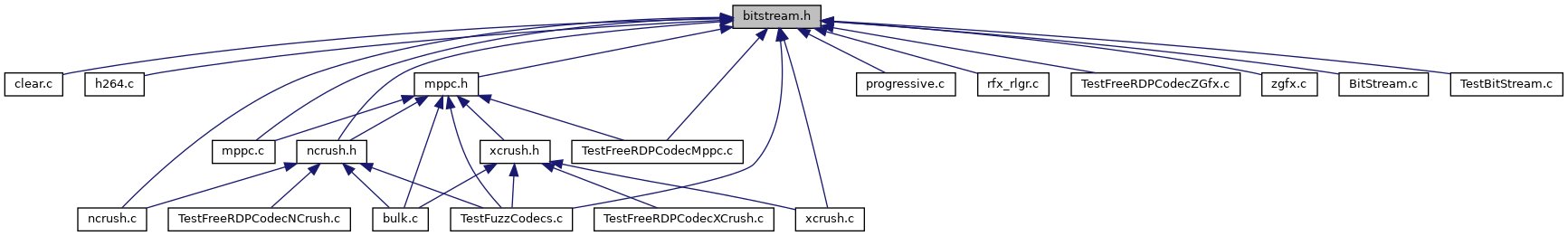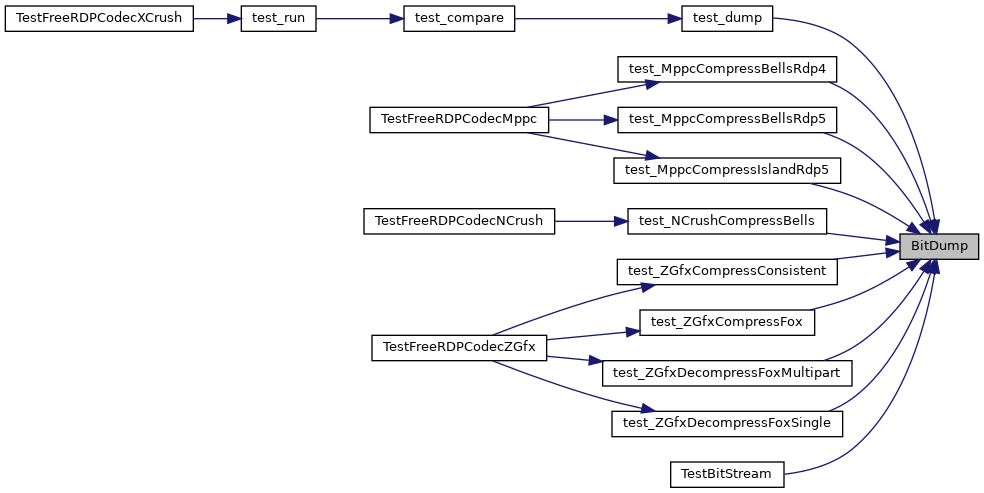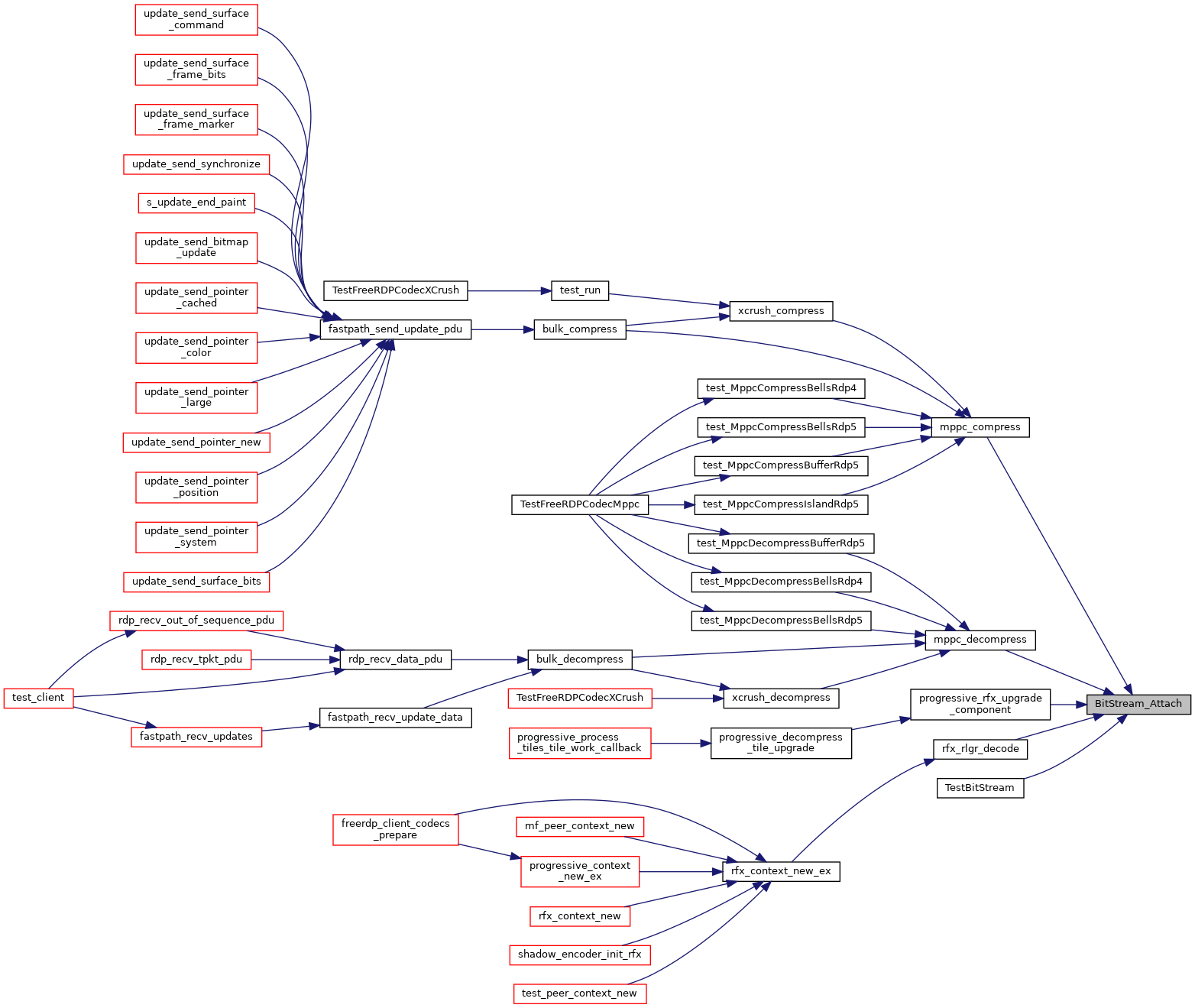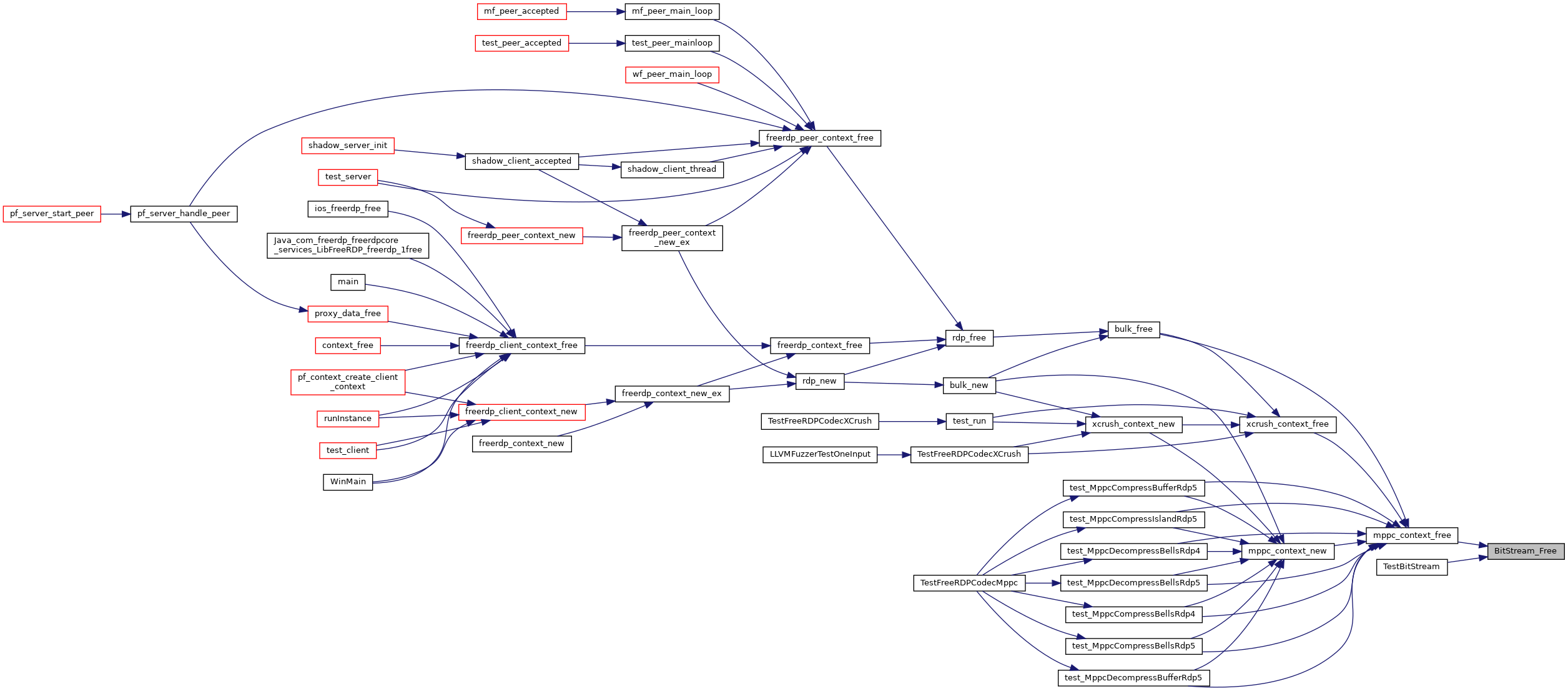FreeRDP
bitstream.h File Reference
`#include <winpr/winpr.h>`
`#include <winpr/wtypes.h>`
`#include <winpr/crt.h>`
`#include <winpr/wlog.h>`
This graph shows which files directly or indirectly include this file:## Data Structures

struct  wBitStream

## Macros

#define BITDUMP_MSB_FIRST   0x00000001

#define BITDUMP_STDERR   0x00000002

#define BitStream_Prefetch(_bs)

#define BitStream_Fetch(_bs)

#define BitStream_Flush(_bs)

#define BitStream_Shift(_bs, _nbits)

#define BitStream_Shift32(_bs)

#define BitStream_Write_Bits(_bs, _bits, _nbits)

#define BitStream_GetRemainingLength(_bs)   (_bs->length - _bs->position)

## Functions

WINPR_API void BitDump (const char *tag, UINT32 level, const BYTE *buffer, UINT32 length, UINT32 flags)

WINPR_API UINT32 ReverseBits32 (UINT32 bits, UINT32 nbits)

WINPR_API void BitStream_Attach (wBitStream *bs, const BYTE *buffer, UINT32 capacity)

WINPR_API wBitStream * BitStream_New ()

WINPR_API void BitStream_Free (wBitStream *bs)

## Macro Definition Documentation

 #define BITDUMP_MSB_FIRST   0x00000001
 #define BITDUMP_STDERR   0x00000002
 #define BitStream_Fetch ( _bs )
Value:
do { \
(_bs->accumulator) = 0; \
if (((UINT32) (_bs->pointer - _bs->buffer) + 0) < (_bs->capacity)) \
(_bs->accumulator) |= (*(_bs->pointer + 0) << 24); \
if (((UINT32) (_bs->pointer - _bs->buffer) + 1) < (_bs->capacity)) \
(_bs->accumulator) |= (*(_bs->pointer + 1) << 16); \
if (((UINT32) (_bs->pointer - _bs->buffer) + 2) < (_bs->capacity)) \
(_bs->accumulator) |= (*(_bs->pointer + 2) << 8); \
if (((UINT32) (_bs->pointer - _bs->buffer) + 3) <(_bs->capacity)) \
(_bs->accumulator) |= (*(_bs->pointer + 3) << 0); \
} while(0)
#define BitStream_Prefetch(_bs)
Definition: bitstream.h:50
winpr freerdp if(WITH_DEBUG_SYMBOLS AND MSVC AND NOT BUILTIN_CHANNELS AND BUILD_SHARED_LIBS) install(FILES \$
Definition: channels/drive/client/CMakeLists.txt:32
typedef UINT32
Definition: wf_interface.h:116
 #define BitStream_Flush ( _bs )
Value:
do { \
if (((UINT32) (_bs->pointer - _bs->buffer) + 0) < (_bs->capacity)) \
*(_bs->pointer + 0) = (_bs->accumulator >> 24); \
if (((UINT32) (_bs->pointer - _bs->buffer) + 1) < (_bs->capacity)) \
*(_bs->pointer + 1) = (_bs->accumulator >> 16); \
if (((UINT32) (_bs->pointer - _bs->buffer) + 2) < (_bs->capacity)) \
*(_bs->pointer + 2) = (_bs->accumulator >> 8); \
if (((UINT32) (_bs->pointer - _bs->buffer) + 3) < (_bs->capacity)) \
*(_bs->pointer + 3) = (_bs->accumulator >> 0); \
} while(0)
winpr freerdp if(WITH_DEBUG_SYMBOLS AND MSVC AND NOT BUILTIN_CHANNELS AND BUILD_SHARED_LIBS) install(FILES \$
Definition: channels/drive/client/CMakeLists.txt:32
typedef UINT32
Definition: wf_interface.h:116
 #define BitStream_GetRemainingLength ( _bs ) (_bs->length - _bs->position)
 #define BitStream_Prefetch ( _bs )
Value:
do { \
(_bs->prefetch) = 0; \
if (((UINT32) (_bs->pointer - _bs->buffer) + 4) < (_bs->capacity)) \
(_bs->prefetch) |= (*(_bs->pointer + 4) << 24); \
if (((UINT32) (_bs->pointer - _bs->buffer) + 5) < (_bs->capacity)) \
(_bs->prefetch) |= (*(_bs->pointer + 5) << 16); \
if (((UINT32) (_bs->pointer - _bs->buffer) + 6) < (_bs->capacity)) \
(_bs->prefetch) |= (*(_bs->pointer + 6) << 8); \
if (((UINT32) (_bs->pointer - _bs->buffer) + 7) < (_bs->capacity)) \
(_bs->prefetch) |= (*(_bs->pointer + 7) << 0); \
} while(0)
winpr freerdp if(WITH_DEBUG_SYMBOLS AND MSVC AND NOT BUILTIN_CHANNELS AND BUILD_SHARED_LIBS) install(FILES \$
Definition: channels/drive/client/CMakeLists.txt:32
typedef UINT32
Definition: wf_interface.h:116
 #define BitStream_Shift ( _bs, _nbits )
Value:
do { \
if (_nbits == 0) { \
} else if ((_nbits > 0) && (_nbits < 32)) { \
_bs->accumulator <<= _nbits; \
_bs->position += _nbits; \
_bs->offset += _nbits; \
if (_bs->offset < 32) { \
_bs->mask = ((1 << _nbits) - 1); \
_bs->accumulator |= ((_bs->prefetch >> (32 - _nbits)) & _bs->mask); \
_bs->prefetch <<= _nbits; \
} else { \
_bs->mask = ((1 << _nbits) - 1); \
_bs->accumulator |= ((_bs->prefetch >> (32 - _nbits)) & _bs->mask); \
_bs->prefetch <<= _nbits; \
_bs->offset -= 32; \
_bs->pointer += 4; \
if (_bs->offset) { \
_bs->mask = ((1 << _bs->offset) - 1); \
_bs->accumulator |= ((_bs->prefetch >> (32 - _bs->offset)) & _bs->mask); \
_bs->prefetch <<= _bs->offset; \
} \
} \
} else { \
WLog_WARN("com.winpr.bitstream", "warning: BitStream_Shift(%u)", (unsigned)_nbits); \
} \
} while(0)
#define BitStream_Prefetch(_bs)
Definition: bitstream.h:50
#define WLog_WARN(tag,...)
Definition: include/winpr/wlog.h:160
winpr freerdp if(WITH_DEBUG_SYMBOLS AND MSVC AND NOT BUILTIN_CHANNELS AND BUILD_SHARED_LIBS) install(FILES \$
Definition: channels/drive/client/CMakeLists.txt:32
 #define BitStream_Shift32 ( _bs )
Value:
do { \
BitStream_Shift(_bs, 16); \
} while(0)
#define BitStream_Shift(_bs, _nbits)
Definition: bitstream.h:86
 #define BitStream_Write_Bits ( _bs, _bits, _nbits )
Value:
do { \
_bs->position += _nbits; \
_bs->offset += _nbits; \
if (_bs->offset < 32) { \
_bs->accumulator |= (_bits << (32 - _bs->offset)); \
} else { \
_bs->offset -= 32; \
_bs->mask = ((1 << (_nbits - _bs->offset)) - 1); \
_bs->accumulator |= ((_bits >> _bs->offset) & _bs->mask); \
_bs->accumulator = 0; \
_bs->pointer += 4; \
if (_bs->offset) { \
_bs->mask = ((1 << _bs->offset) - 1); \
_bs->accumulator |= ((_bits & _bs->mask) << (32 - _bs->offset)); \
} \
} \
} while(0)
#define BitStream_Flush(_bs)
Definition: bitstream.h:75
winpr freerdp if(WITH_DEBUG_SYMBOLS AND MSVC AND NOT BUILTIN_CHANNELS AND BUILD_SHARED_LIBS) install(FILES \$
Definition: channels/drive/client/CMakeLists.txt:32

## Function Documentation

 WINPR_API void BitDump ( const char * tag, UINT32 level, const BYTE * buffer, UINT32 length, UINT32 flags )

Here is the caller graph for this function:WINPR_API void BitStream_Attach ( wBitStream * bs, const BYTE * buffer, UINT32 capacity )

Here is the caller graph for this function:WINPR_API void BitStream_Free ( wBitStream * bs )

Here is the caller graph for this function:WINPR_API wBitStream* BitStream_New ( )

Here is the caller graph for this function:WINPR_API UINT32 ReverseBits32 ( UINT32 bits, UINT32 nbits )